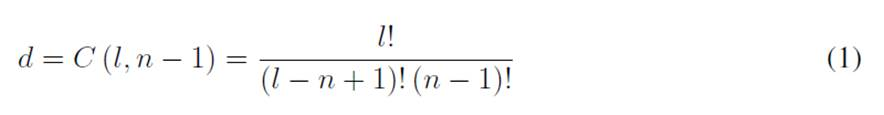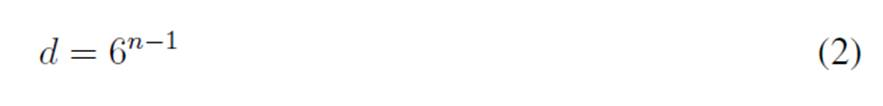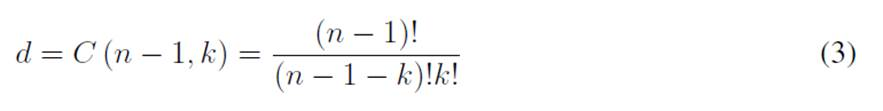2022-04-25

Editorial

# Notes on the Dimension of the Solution Space in Typical Electrical Engineering Optimization Problems

## Authors

• Oscar Danilo Montoya Universidad Distrital Francisco José de Caldas

* (en).

## Author Biography

### Oscar Danilo Montoya, Universidad Distrital Francisco José de Caldas

Docente Asistente, Facultad de Ingeniería, Proyecto curricular de Ingeniería Eléctrica Universidad Distrital Francisco José de Caldas, Bogotá D.C., Colombia. Docente Investigador, Laboratorio Inteligente de Energía Universidad Tecnológica de Bolívar, Cartagena, Colombia.

126

## PlumX

Nowadays, optimization methodologies based on combinatorial strategies (i.e., metaheuristic methods) and exact methods can be easily found through out the scientific literature in all areas of engineering, including electrical, mechanical, chemical, computational, and food engineering, among others. The common denominator in these areas of research corresponds to the complexity of the optimization models, as well as to the large dimensions of the solution space where these models are defined 1. In addition, most of these models combine binary (also integer) decision variables with continuous ones into nonlinear non-convex formulations, which complicates the application of exact solution methods.

Electrical engineering does not escape these problems; on the contrary, many of its optimization problems are defined on the family of mixed-integer nonlinear programming models 2. Three of the most common optimization problems in electrical engineering are: (i) the reconfiguration of primary distribution feeders 3; (ii) phase-balancing in three-phase distribution grids 4; and (iii) the location of shunt devices (i.e., dispersed generation, capacitor banks or static compensators) in distribution networks 5.

The solution to these problems in electrical engineering contributes to improving the quality of the electricity service provided by distribution companies to all end-users, given that their objective functions are typically the minimization of the total grid energy losses 6. Moreover, these are connected with the concept of energy efficiency due to the fact that energy loss minimization is directly linked to the reduction of power generation, which, in the case of the fossil fuels, implies minimizing greenhouse gas emissions from the electrical sector 7. Note that the aforementioned electrical engineering problems combine power flow equations (nonlinear and non-convex due to products among voltajes with trigonometric functions) with binary and integer variables that make their solution with exact optimization methodologies a much more complex issue 8. Owing to the combination of the discrete and continuous variables in an MINLP model, in the scientific literature, the solution of these models is typically carried out with master-slave methodologies based on metaheuristics 9. The master stage is entrusted with solving the discrete problem, and the slave stage solves the continuous component of the model. However, prior to applying these solution methodologies, it is necessary to determine the size of the solution space of the optimization problem’s discrete component since this dimension will define whether there is a need to use combinatorial optimizers in the solution.

## Reconfiguration of primary distribution feeders

The problem regarding the reconfiguration of primary distribution feeders consists of selecting a set of distribution lines that must be operated to minimize the total grid power losses for a typical demand condition. To illustrate this problem, Fig 1 presents the electrical configuration of the IEEE 33-bus system for reconfiguration studies 10.

The main challenge in the distribution feeder reconfiguration for a grid with n nodes is to find the radial configuration of the system, i.e., a set composed of n − 1 distribution lines that minimizes the total losses in all the branches of the network. Note that, in this problem, that the number of available paths l to be selected is higher than the number of nodes, which implies that all the possible solutions for this problem are defined by the combination C (l, n − 1), i.e.:

Also note that, if Eq. (1) is applied to the test feeder in Fig. 1, then the dimension of the solution space for this problem is 435897.## Phase-balancing in three-phase distribution grids

The phase-balancing problem in three-phase distribution networks corresponds to determining the type of connection of the load at each node of the network from six possible options, namely from sequence ABC (type 1) to sequence CBA (type 6) 4. To determine the size of the solution space for the problem regarding phase-balancing in distribution grids, let us consider the test feeder presented in Fig. 1, which has n = 33 nodes. Thereupon, phase balancing is applied to all nodes except the substation bus. For this reason, the size of the solution space, i.e., d, is defined as follows:which, for the IEEE 33-bus system, will be equal to 7958661109946400884391936, i.e., the dimensión of the solution space has about 7:9586611099464 x 1024 possible solutions.

## Location of shunt devices

The problem regarding the location of shunt devices in distribution grids implies exploring the possibility of integrating distributed energy resources in the networks to reduce energy losses, annual operative costs, or improving voltage profile performance, among other objectives 5 If there are k shunt devices available for installation and the system is composed of n − 1 nodes (except the substation bus), then the solution of the problem is to identify the best k nodes from the total n. This allows minimizing the objective function under study. In this context, the dimension of the solution space can be defined as follows 9:

Note that, if Eq. (3) is applied to the test feeder in Fig. 1 with k = 3, then the dimension of the solution space is 4960. However, if k is selected as 5, the dimension of the solution space increases to 201376.General commentaries

The analysis of the dimension of the solution space in the three studied problems is an important step prior to the selection of an optimization technique in order to deal with their solutions. Note that, in all cases, the dimension of the solution space depends on the size of the distribution network, as well as on the number of elements that will be included in their analysis, which define the dimensions of the solution space from thousands to billions of options including enormous solution spaces, as is the case of the phase-balancing problem.

On the other hand, for the field of electrical engineering, the solution of the studied problems is of the utmost importance since they are related to potential improvements in the energy service provided by distribution companies. This includes, for instance, the minimization of the total grid energy losses (i.e., improving the efficiency of the electrical energy service). These issues are connected with the reduction of the greenhouse gas emissions from fossil sources used to generate electricity, which is nowadays society’s main objective for the XXI century.

## References

 J. B. Odili, “Combinatorial optimization in science and engineering,” Curr. Sci., vol. 113, no. 12, p. 2268, Dec. 2017.

 M. Lavorato, J. F. Franco, M. J. Rider, and R. Romero, “Imposing radiality constraints in distribution system optimization Problems,” IEEE Transact. Power Syst., vol. 27, no. 1, pp. 172-180, Feb. 2012.

 J. A. Taylor and F. S. Hover, “Convex models of distribution system reconfiguration,” IEEE Transact. Power Sys., vol. 27, no. 3, pp. 1407-1413, Aug. 2012.

 K. Ma, L. Fang, and W. Kong, “Review of distribution network phase unbalance: scale, causes, consequences, solutions, and future research directions,” CSEE J. Power Energy Sys., vol. 6, no. 3, pp. 479-488, 2020.

 S. Kaur, G. Kumbhar, and J. Sharma, “A MINLP technique for optimal placement of multiple DG units in distribution systems,” Int. J. Elec. Power Energy Sys., vol. 63, pp. 609-617, Dec. 2014.

 G. Srinivasan, “Optimization of distributed generation units in reactive power compensated reconfigured distribution network,” Automatika, vol. 62, no. 2, pp. 249-263, Apr. 2021.

 P. A. Owusu and S. Asumadu-Sarkodie, “A review of renewable energy sources, sustainability issues and climate change mitigation,” Cogent Eng., vol. 3, no. 1, p. 1167990, Apr. 2016.

 D. K. Molzahn, “Identifying and characterizing non-convexities in feasible spaces of optimal power flow problems,” IEEE Transact. Circ. Sys. II: Express Briefs, vol. 65, no. 5, pp. 672-676, May 2018.

 O. D. Montoya, “A convex OPF approximation for selecting the best candidate nodes for optimal location of power sources on DC resistive networks,” Eng. Sci. Tech. Int. J., vol. 23, no. 3, pp. 527-533, Jun. 2020.

 V. Vai, S. Suk, R. Lorm, C. Chhlonh, S. Eng, and L. Bun, “Optimal reconfiguration in distribution systems with distributed generations based on modified sequential switch opening and exchange,” App. Sci., vol. 11, no. 5, p. 2146, Feb. 2021.

Montoya O. D., “Notes on the dimension of the solution space in typical electrical engineering optimization problems”. Ing., vol. 27, no. 2, 2022. e19310